The search for  new physics.

 Some Notes on Understanding the Schrodinger Wave Equation   D.M. Marett 2006    Some Useful Resources: The Orbitron - An excellent website for modelling orbitals       How did Schrodinger arrive at his wave equation? This is a short mathematical exercise to get to the root of what the equation is really describing, and how to go through the math step by step to derive information about the simplest of the atoms, hydrogen. In order to do this, we start with an equation to describe a plane wave moving through space. In 2D, a point at x on the x and y axis, which has a plane wave moving through it, will oscillate up and down with the peaks and troughs. The amplitude of x (in units of y) will then obey the travelling wave equation: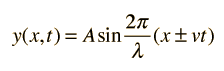Where for a fixed position y, as time passes (t changes) for a fixed velocity v, and maximum amplitude A, the value of y will change sinusoidally reflecting the up and down movement of point x. See the Wikipedia article here or the Hyperphysics explanation here for more detail.     Schrodinger knew that de Broglie had described an electron as a wave moving around the nucleus. Thus Shrodinger tried to model what would happen if a wave moved around the nucleus, such as a travelling wave. He used the equation: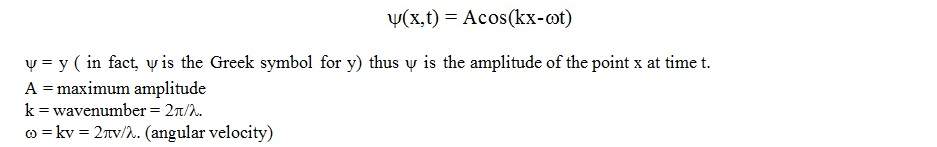For the point x independent of time, he used (Ref1):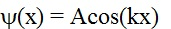Now, Shrodinger needed to somehow equate the energy of the electron as a particle, with the energy of a travelling wave. If he could do this, then he could substitute the travelling wave equation into Bohr’s equations for the motion of an electron around the nucleus, and model the particle as a wave.   Shrodinger knew that: 1) The total energy of an electron = its kinetic energy + its potential energy.   KE + PE = E total                  KE = 1/2mv2.   2) That the wavelength he wanted was the de Broglie wavelength, that could be expressed as: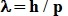where p = momentum 3) The potential energy of an electron in a hydrogen atom is:   U =  kQ q                where: k = coulombs constant, 9 x 10 9 N               r                                 Q is the charge of the electron                                                 q = charge of the proton                                                    r = radius           = 13.61eV x 2   To equate the kinetic energy of the particle to the energy of the wave, Schrodinger had to use an expression for kinetic energy that included the wavenumber k.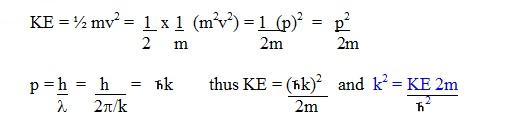Having written the kinetic energy of the particle in terms of k, he now had to write the travelling wave equation in terms of k as well (Ref1).   The travelling wave equation, independent of time was:      y(x) = Acos(kx)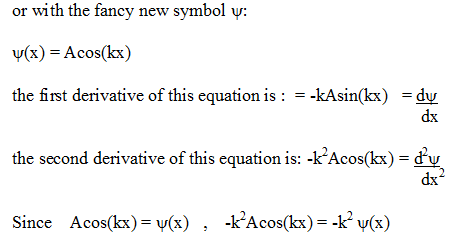This is just basic calculus. The use of the second derivative   d2y in describing travelling                                                                                                                          dx2 waves is also shown in the Hyperphysics reference here.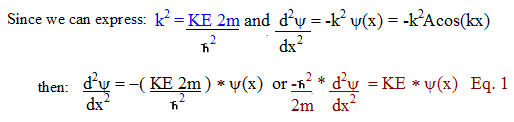Now Schrodinger had an equation to express the travelling wave in terms of the kinetic energy of the electron around the nucleus. However, he wanted to be able to include the potential energy in the equation, since this value was usually the only known value.   To do this, he had to substitute the KE value for a value including the potential energy U.   KE + U = E total,   thus KE = E-U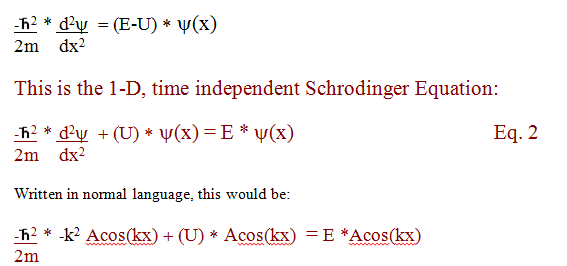The constant A is usually determined by evaluating or setting boundary conditions.   For an electron and a proton, the U force between them is:  -e2                                                                                         4peo r We also need to determine the second derivative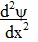of the distance r in spherical coordinates.                                                                                                                                           This is: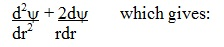At Ref1 it is explained how this equation is then applied to the situation of a real atom, such as hydrogen:   For the case of the hydrogen atom: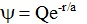where Q and a are constants.   The first derivative of this expression with respect to r is :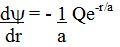The second derivative of this expression with respect to r is :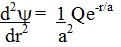How the equation for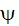was determined is not shown.   For the hydrogen atom, a = 1 Bohr radius and Q =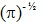=e –r/ 5.29E-11m   How do Imaginary Numbers come into the Schrodinger Equation?   We start with the 1D time independent equation:For the time-dependent form of the equation, it is stated that: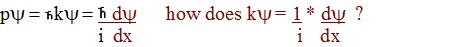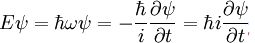To understand how imaginary numbers are used to describe waves, we need to understand  Euler’s formula and how it is applied to plane wave equations:   Euler’s Formula: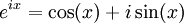A wave moving in the x-direction has the form (the general form of the wave function):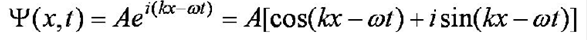Ref: http://www.mines.edu/Academic/courses/physics/phgn300/Sarazin/PDFs/QuantumLect4.pdf      p. 3.                                                                                                             Basically, one wants to know how the imaginary number i gets introduced into the Schrodinger equation. Start with the equality:    py = hky =  h    dy                                            2p    2p i dx                                  thus:      ky =  1  * dy                                                i    dx                           The obvious question is, how does the i get into the equation if y= ACos(kx)?                              After checking the site http://en.citizendium.org/wiki/Schr%C3%B6dinger_equation, they referenced another site at: http://walet.phy.umist.ac.uk/QM/LectureNotes/ under "The Schrodinger Equation - the state of the quantum system" where one finds the equality used again. And here is the trick - now, rather than using y=ACos(kx) to describe the planewave (1D), they use the equivalent expression for a plane wave from Euler’s formula:                              Aeix= A[cos(x) + isin(x)] where x = kx (for 1D) or kx-wt (for 2D)   Thus y=Aei(kx) which describes a plane wave (1D) as well as y=ACos(kx) but is complex.   The derivative of Aei(kx) is:  dy = ikAei(kx)                          dx                                Thus ky =  1  * dy   =  1 * ikAei(kx)  = k * Aei(kx)  = ky .                                                                                             i     dx         i                                 So that is the answer - they use y=ACos(kx) to develop the first part of the 1D Shrodinger equation, and then when they want to make the equation time dependent y(x,t), they introduce complex numbers using the alternative plane wave expression                                                                                              y= Aei(kx-wt).             In the end, the Schrodinger equation doesn't seem as difficult if one understands that rather than moving around simple variables to make things add up, instead they introduce the derivatives of variables to make them add up. Unfortunately, this leads to a partial differential equation that is very hard to solve.   Rather than  solving the partial differential equation arising in the 1D Schrodinger equation, it appears that the answer is usually arrived at by “guessing” that the the wavefunction corresponds to a form:. This is the associated Laguerre function for 1s hydrogen.   From this guess, one works backwards. See: http://spiff.rit.edu/classes/phys314/lectures/sch_hyd/sch_hyd.html http://www.physics.gatech.edu/gcuo/lectures/ModernPhysicsLectures/MP16HydrogenAtom.ppt#11 http://phy.asu.edu/phy361-alarcon/SPRING07/lecture20_0405.ppt#11 Where it is always taught the same.   The derivatives of this associated Laguerre function are then inserted into the equation:which are then inserted into equation 3 below:Eq. 3 then becomes:Since two terms contain 1/r * e-r/a , and two terms contain only e-r/a , for the equation to work at all values of r, the terms with the same variables have to equal each other. Thus: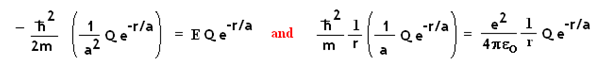Cancelling out the common terms on both sides of the equations, a and E are easily solved for the hydrogen atom:   a = 5.29 E -11 m,         E= 2.18E-18 J (13.61 eV)     ******